# Abelian group

(diff) ← Older revision | Latest revision (diff) | Newer revision → (diff)
Jump to: navigation, search

A group whose operation is commutative (cf. Commutativity). They are named after N.H. Abel, who used such groups in the theory of solving algebraic equations by means of radicals. It is customary to write the operation in an Abelian group in additive notation, i.e. to use the plus sign () for that operation, called "addition" , and the zero sign

for the neutral element, named zero (in multiplicative notation it is called the unit element).

Examples of Abelian groups. All cyclic groups (cf. Cyclic group) are Abelian, in particular, the additive group of integers. All direct sums (cf. Direct sum) of cyclic groups are Abelian. Also the additive group of rational numbers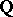is Abelian; it is moreover a locally cyclic group, i.e. a group in which all finitely generated subgroups are cyclic. Finally, the groups of type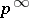(or the quasi-cyclic groups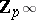), whereis an arbitrary prime number, are Abelian (cf. Group of type; Quasi-cyclic group).

The free composition in the variety of Abelian groups coincides with the direct sum. A free Abelian group is a direct sum of infinite cyclic groups. Every subgroup of a free Abelian group is free Abelian. The set of all elements of finite order in an Abelian group forms a subgroup, which is called the torsion subgroup (periodic part) of the Abelian group. The quotient group of an Abelian group by its torsion subgroup is a group without torsion. Thus, every Abelian group is an extension of a torsion-free group by a torsion group. This extension does not always split, i.e. the torsion group is usually not a direct summand. An Abelian torsion group in which the order of every element is a power of a fixed prime numberis said to be primary with respect to(the term-group is used in general group theory). Every torsion group splits uniquely into a direct sum of primary groups that correspond to distinct prime numbers.

A complete classification is known for finitely generated Abelian groups. This is given by the fundamental theorem of finitely generated Abelian groups: Every finitely generated Abelian group is a direct sum of finitely many non-split cyclic subgroups some of which are finite and primary, while the others are infinite (G. Frobenius, L. Stickelberger). In particular, finite Abelian groups split into a direct sum of primary cyclic groups. Such splittings are, in general, not unique, but any two splittings of a finitely generated Abelian group into direct sums of non-split cyclic groups are isomorphic, so that the number of infinite cyclic summands and the collection of the orders of the primary cyclic summands do not depend on the splittings chosen. These numbers are called invariants of the finitely generated Abelian group. They constitute a complete system of invariants, in the sense that two (finitely generated) Abelian groups are isomorphic if and only if they have the same invariants. Each subgroup of a finitely generated Abelian group is itself finitely generated.

Not every Abelian group is a direct sum of (even infinitely many) cyclic groups. For primary groups there is a necessary and sufficient condition for the existence of such splittings, the so-called Kulikov criterion. Letbe a primary Abelian group for a prime number. A non-zero elementofis said to be an element of infinite height inif for any integerthe equation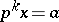is solvable in, andis called an element of heightif this equation is solvable foronly. The Kulikov criterion states that a primary Abelian groupis a direct sum of cyclic groups if and only if it is the union of an increasing sequence of subgroups such that the set of heights of the elements in each one of these subgroups is bounded. Any subgroup of an Abelian group that is a direct sum of cyclic groups itself is such a direct sum. The indecomposable (non-splittable) into direct sums primary Abelian groups are the primary cyclic groups and the group.

A finite set of elements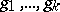in an Abelian group is called linearly dependent if there exist integers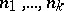, not all equal to zero, such that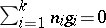. If such numbers do not exist, the set is said to be linearly independent. An arbitrary collection of elements ofis said to be linearly dependent if there exists a finite linearly dependent subcollection. An Abelian group that is not a torsion group has maximal linearly independent sets. The cardinality of all maximal linearly independent collections of elements is the same and is called the rank (Prüfer rank) of the given Abelian group. The rank of a torsion group is considered to be zero. The rank of a free Abelian group coincides with the cardinality of a set of free generators of it.

Every torsion-free Abelian group of rank 1 is isomorphic to some subgroup of the additive group of rational numbers. There exists a complete description of such groups in the language of types. Each element of an Abelian group without torsion determines a characteristic, which is a countable sequence consisting of non-negative numbers and the symbol. This sequence is constructed in the following manner. Suppose that the prime numbers are enumerated in increasing order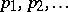. An elementdetermines the sequence whose-th element is equal toif the equation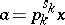is solvable in the group but the equation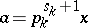is not solvable, and the-th element is equal toif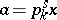is solvable for all. Two characteristics are equivalent if they are equal except possibly for a finite number of places and if the symboloccurs in exactly the same places in both sequences. The characteristics of two linearly dependent elements are equal. A class of equivalent characteristics is said to be a type. To each torsion-free Abelian group of rank 1 there corresponds a uniquely determined type, called the type of the given group; non-isomorphic groups having different types.

A torsion-free Abelian group that splits into a direct sum of groups of rank 1 is said to be completely split. Not every subgroup of a completely split group is completely split, though every direct summand is. For each integerthere exists a torsion-free Abelian group of rankthat cannot be decomposed (or split) into a direct sum. For countable torsion-free Abelian groups one can to construct a complete system of invariants.

An Abelian group is called complete, or divisible, if for any one of its elementsand for any integerthe equation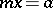has a solution in the group. All divisible Abelian groups turn out to be direct sums of groups isomorphic toand the groups, and the cardinalities of the sets of components isomorphic to, as well as to(for each), from a complete and independent system of invariants of the divisible group. Every Abelian groups can be isomorphically imbedded in some divisible Abelian group. The divisible Abelian groups and only they are the injective objects in the category of Abelian groups. A divisible Abelian group is a direct summand of each Abelian group containing it. Therefore, an Abelian group is a direct sum of a divisible Abelian group and a so-called reduced group, i.e. a group that contains no non-trivial divisible subgroups. A classification of reduced Abelian groups is known only in certain special cases. Thus, Ulm's theorem () gives the classification of all countable reduced Abelian torsion groups.

The theory of Abelian groups has its origins in number theory, and is now extensively applied in many modern mathematical theories. Thus, the duality theory of characters for finite Abelian groups has been considerably extended to the duality theory of locally compact Abelian groups. The development of homological algebra has made it possible to solve a whole series of problems in Abelian groups, such as classifying the set of all extensions of one group by another. The theory of modules is closely connected with Abelian groups regarded as modules over the ring of integers. Many results in the theory of Abelian groups can be applied to the case of modules over a principal ideal ring. Owing to their relative simplicity and to the fact that they have been very thoroughly studied (which is confirmed, for instance, by the solvability of the elementary theory of Abelian groups), and to the availability of a sufficient variety of objects, Abelian groups serve as a constant source of examples in various fields of mathematics.

How to Cite This Entry:
Abelian group. Encyclopedia of Mathematics. URL: http://encyclopediaofmath.org/index.php?title=Abelian_group&oldid=12618
This article was adapted from an original article by Yu.L. Ershov (originator), which appeared in Encyclopedia of Mathematics - ISBN 1402006098. See original article